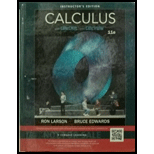Chapter 8, Problem 2PS### Calculus (MindTap Course List)

11th Edition
Ron Larson + 1 other
ISBN: 9781337275347

#### Solutions

Chapter
Section### Calculus (MindTap Course List)

11th Edition
Ron Larson + 1 other
ISBN: 9781337275347
Textbook Problem

# Proof(a) Evaluate the integrals ∫ a 1 ln x   d x   and   ∫ a 1 ( ln x ) 2 d x . (b) Prove that ∫ a 1 ( ln x ) n d x = ( − 1 ) n n ! for all positive integers n.

(a)

To determine

To calculate: The integrals 01lnxdx and 01(lnx)2dx.

Explanation

Given:

The integrals 01lnxdx and 01(lnx)2dx.

Formula used:

The integral formula is

xndx=xn+1n+1

Use the following to do Integration by parts.

fg=fgfg

Calculation:

Solve for 01lnxdx

Use by part integration.

Assuming f=lnx and g=1, we get

f=1x and g=x.

So, the integral now becomes

01lnxdx=xlnx1xxdx=xlnxx

Solving further, we get

01lnxdx=limb0+b1lnxdx=limb0+[xlnxx]b1=(1)limb0+(blnbb)=1

Hence, the value of the integral is 1

(b)

To determine

To prove: 01(lnx)ndx=(1)nn! for all positive integers n.

### Still sussing out bartleby?

Check out a sample textbook solution.

See a sample solution

#### The Solution to Your Study Problems

Bartleby provides explanations to thousands of textbook problems written by our experts, many with advanced degrees!

Get Started

## Additional Math Solutions

#### Find more solutions based on key concepts